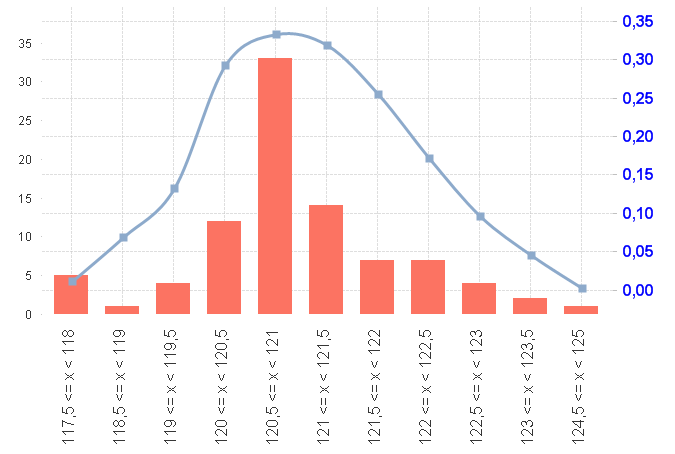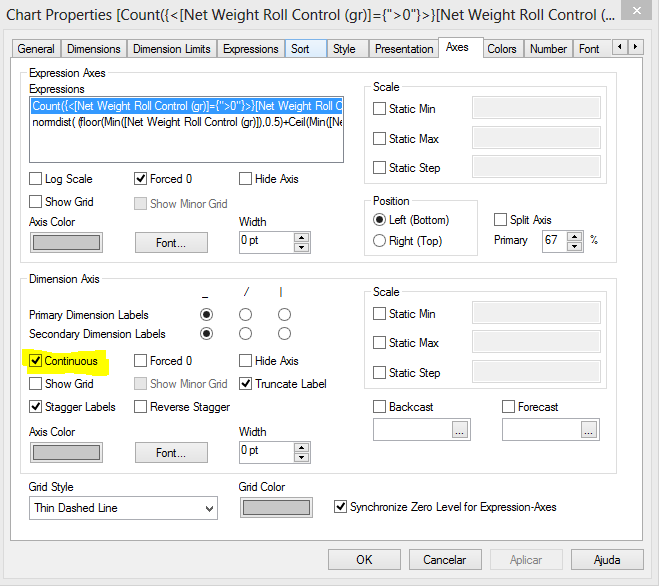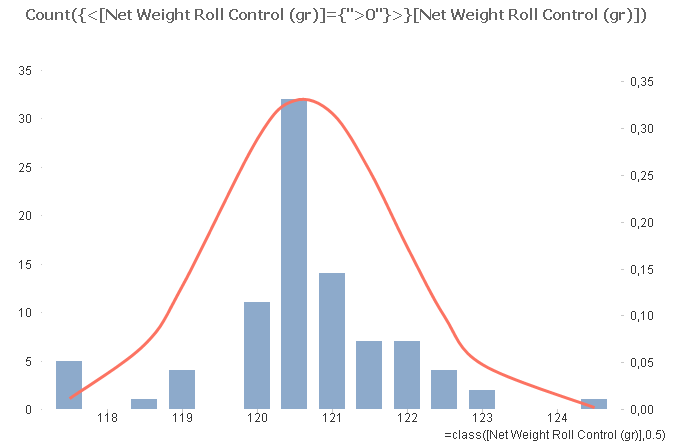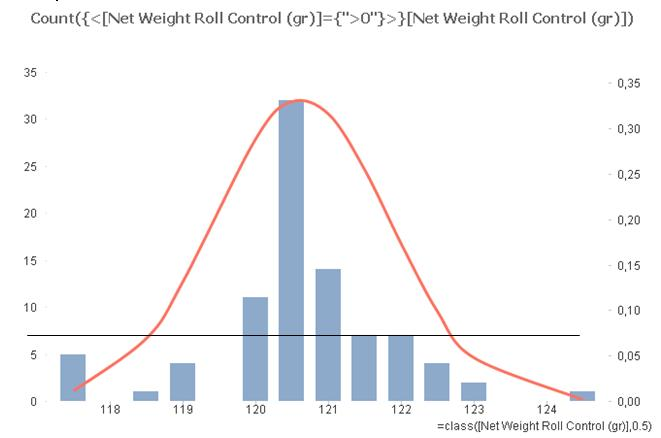QlikView Scripting

Discussion Board for collaboration on QlikView Scripting.

Highlighted
Contributor

Distribution normal

Hello everybody,

I have a question about the normal distribution.

I can not get a chart completly simetric what it is the usual in this kind of chart:The formuls are:

Dimensions: class([Net Weight Roll Control (gr)],0.5)

Expressions:

normdist( (floor(Min([Net Weight Roll Control (gr)]),0.5)+Ceil(Min([Net Weight Roll Control (gr)]),0.5))/2,

avg({1} total {<[Net Weight Roll Control (gr)]={">0"}>} [Net Weight Roll Control (gr)]),

stdev({1} total {<[Net Weight Roll Control (gr)]={">0"}>} [Net Weight Roll Control (gr)]),0)

Count({<[Net Weight Roll Control (gr)]={">0"}>}[Net Weight Roll Control (gr)])

What I try with: floor(Min([Net Weight Roll Control (gr)]),0.5)+Ceil(Min([Net Weight Roll Control (gr)]),0.5))/2 is to fix the medium of each interval to draw the distribution. In theory, it must be simetric, but follows the form got in the frecuency formul.

I attached the excell file.

Please, don´t send me the answer in qlik examples, because i have edition personal.

Tags (2)
7 RepliesMVP

Re: Distribution normal

That's because your x-axis is not continuous. Have a close look to the intervals shown at the axis, you'll notice that some intermediate intervals are missing (e.g.118 - 118.5).

If you use a continuous axis on axis tab, the curve should look much more as you expect it.Employee

Re: Distribution normal

Using swuehl recomendation

Contributor

Re: Distribution normal

Hi Clever:

But I am usign Persoanl Edition, therefore I can not open the file.

Could you explain what is your solution with formuls?Employee

Re: Re: Distribution normal

I´ve used an expression (and marked as Line/Smooth)

normdist( (floor(Min([Net Weight Roll Control (gr)]),0.5)+Ceil(Min([Net Weight Roll Control (gr)]),0.5))/2,

avg({1} total {<[Net Weight Roll Control (gr)]={">0"}>} [Net Weight Roll Control (gr)]),

stdev({1} total {<[Net Weight Roll Control (gr)]={">0"}>} [Net Weight Roll Control (gr)]),0)

I marked "continuous" into Axes TabEmployee

Re: Distribution normal

normdist( Count({<[Net Weight Roll Control (gr)]={">0"}>}[Net Weight Roll Control (gr)]),

avg({1} total {<[Net Weight Roll Control (gr)]={">0"}>} [Net Weight Roll Control (gr)]),

stdev({1} total {<[Net Weight Roll Control (gr)]={">0"}>} [Net Weight Roll Control (gr)]),0)

gives almost the same curve

Contributor

Re: Distribution normal

Hi Clever:

Thanks for your answer but the chart is not completely simetric. I have attached your chart and my chart where is more evident:The problem is the lack of values in the midle of the intervals.

For solving this problem is necessary to solve this lack.MVP

Re: Re: Distribution normal

I think you won't necessarily get a perfect symmetric curve because you are using discrete sampling points.

You can get a smoother, more symmetric curve if you create additional dimension values so your line curve gets calculated for more sampling points:

// Script to read your data and create a complete range of dimension values (adapt 115 start value and 20 sampling

// points to your range

LOAD class(115+recno()/2,0.5) as Class

AutoGenerate 20;

LOAD [Net Weight Roll Control (gr)],

class([Net Weight Roll Control (gr)],0.5) as Class

FROM

[Net Values Weight.xlsx]

(ooxml, embedded labels, table is Sheet1);

Then use a dimension Class and as expression for the line

=normdist( Class,

avg({1} total {<[Net Weight Roll Control (gr)]={">0"}>} [Net Weight Roll Control (gr)]),

stdev({1} total {<[Net Weight Roll Control (gr)]={">0"}>} [Net Weight Roll Control (gr)]),0)